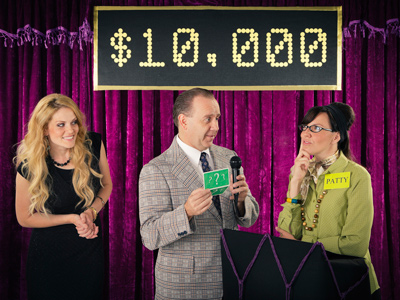In Spanish, where would you put punctuation marks in numbers? That's the question!

# Math - Punctuation Marks with Numbers

This Spanish Difficult Review quiz will take a look at the usage of punctuation marks with numbers as they appear in Spanish. In English, when we get to higher numbers, beginning in the thousands, we place a comma after the thousands and after the millions and after the billions and so on. In Spanish the comma is not used. Rather, a period is used.

Let’s look at an example. In English we would write the following number as: 2,431. In Spanish that number would be written as: 2.431. The period is not the same thing as the English decimal point so do not confuse this with the number.

In addition, in English we use the decimal point to reflect cents or percentages. For example: \$5,555.25. In Spanish, the comma is used where the decimal point is used so this same number in Spanish would be written as: \$5.555,25. It is the exact opposite of English. With a little practice, however, you should be able to quickly write out numbers in both English and in Spanish. Therefore, for this quiz you will be given ten numbers as they would be written in English. Your task is to find the correct Spanish form for that same number.

1.
3,495,789
3,495,789
3.495.789
3,495.789
3.495.78,9
There is no decimal shown in the given number. The last answer improperly shows a place for a decimal. The first answer repeats the English number which is not correct in Spanish. The third answer improperly uses a comma after the 3. The second answer shows the complete, correct Spanish form.
2.
1,111.95
1.111,95
1111.95
1111,95
1.111.95
In Spanish the decimal point is replaced with a comma. The second and last answers have not replaced the decimal. The third answer is missing the period after the first 1. The first answer has correctly replaced both punctuation marks.
3.
89,543,234,170
89.543234170
89.543.234,170
89.543.234.170
89,543.234.170
The first answer is missing several punctuation marks. The second answer used the mark for a decimal but there is no such mark in the English number. The last answer improperly put a comma after 89. The third answer shows each mark correctly used in Spanish.
4.
5.987
5987
.5987
59.87
5,987
The English number contains a decimal which, in Spanish, will become a comma. The first answer is completely missing any punctuation. The second and third answers contain improperly placed punctuation marks and not the correct marks to be used. The last answer shows the correct decimal usage in Spanish.
5.
\$4,672.10
4.672,10\$
\$4.672.10
\$4.672,10
\$4,672,10
The first answer shows the correct usage of the punctuation marks in Spanish but has improperly put the dollar sign at the end. The second answer does not show where a decimal should be and the last answer shows where two decimals should be. Both are not correct. The third answer shows the proper usage of punctuation marks in Spanish. (As a side note here, although we mentioned that putting the dollar sign at the end was improper, we do want to point out that, in some Spanish speaking regions, they will actually put the dollar symbol at the end.)
6.
1
.1
1
,1
1.0
This one might seem a little tricky at first but it is really simple. As there are no punctuation marks in the English number, there shouldn’t be any in the Spanish either. The second answer is the correct and as it shows no punctuation marks.
7.
333,333,333,333.333
333.333.333.333,333
333.333.333,333.333
333.333,333.333,333
333,333,333,333.333
The last answer is the same number as the given number so it is not the correct Spanish form. Wherever there is a comma in English there should be a period in Spanish and wherever there is a period (decimal point) in English there should be a comma in Spanish. The only answer showing this is the first answer.
8.
00.71
71
71,00
71.00
00,71
The given English answer shows a punctuation mark so one should appear in the answer. The first answer has no punctuation mark. The second and third answers have changed the order of the numbers making them different numbers altogether. The last answer shows the number correctly written in Spanish.
9.
\$65,000.00
\$65.000.00
\$65,000.00
\$65.000,00
65.000,00
The last answer has lost the symbol for money which means it is incorrect. The first answer does not show where the Spanish decimal would be. The second answer is the same number as the given number – not the Spanish form. The third answer shows the correct Spanish usage of the punctuation marks.
10.
13.006987
13,006,987
13,006987
13.006,987
13006987
As the given English number shows a punctuation, so too must the answer. The last answer has no punctuations making it incorrect. The first answer shows where two decimal points would be which is not correct. The third answer improperly retained the period and put a decimal point in as well. That, too, is not correct. The second answer shows the correct Spanish punctuation where the decimal point is in English.
Author:  Christine G. Broome